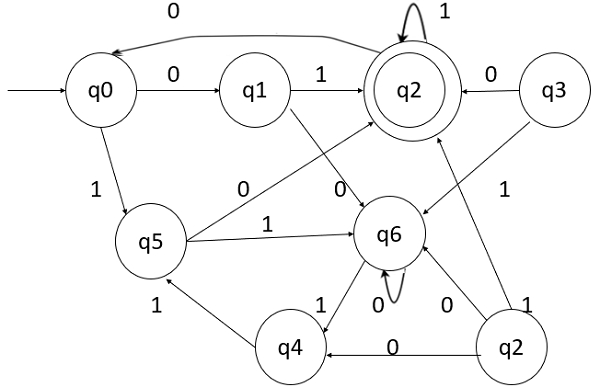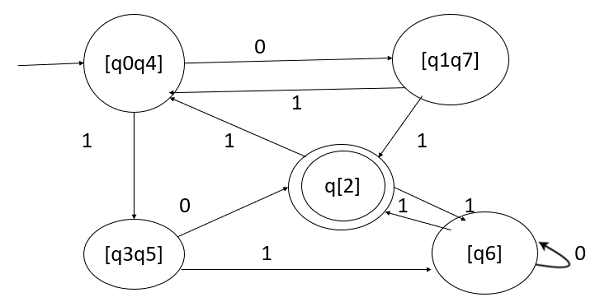# Construct the minimum DFA for any given finite automata.

## Problem

Construct a minimum state DFA for the following automata −## Solution

We first construct a transition table for the given finite automata −

States\inputs01
q0q1q5
q1q6q2
*q2q0q2
q3q2q6
q4q7q5
q5q2q6
q6q6q4
q7q6q2

Q={q0,q1,q2,q3,q4,q5,q6,q7}

Q01={q2} and Q02={q0,q1,q2,q3,q4,q5,q6,q7}

S0={{q2} {q0,q1,q2,q3,q4,q5,q6,q7}}

Consider the set {q0,q1,q2,q3,q4,q5,q6,q7}

{q2} {q0,q1,q3,q5,q6,q7}

{q2} {q0,q4,q6} {q1,q3,q5,q7}

{q2} {q0,q4} {q6} {q1,q3,q5,q7}

{q2}{q0,q4}{q6}{q1,q7}{q3,q5}

The minimized state is as follows −

M1=(Q1, Σ, δ1,q01,F1)

Q1= {[q2],[q0,q4],[q6],[q1,q7],[q3,q5]}

qo1= {[q0,q4]}

F1= {[q2]}

## Transition Table

Now the transition table is as follows −

States\inputs01
[q0,q4][q1,q7][q3,q5]
[q6][q6][q2]
[q1,q7][q0,q4][q2]
[q3,q5][q2][q6]
[q2][q6][q0,q4]

The transition diagram is as follows −